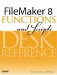# Exact()

Category: Text

 Syntax: Exact ( originalText; comparisonText )

Parameters:

originalTextAny text expression, text field, or container field.

comparisonTextAny text expression, text field, or container field.

Data type returned: Number

Description:

Compares the contents of any two text or container expressions. This function is case sensitive. If the expressions match, the result is 1 (True); otherwise, the result is 0 (False). For container fields, not only must the data be the same, but it must also be stored in the same manner (either embedded or stored by file reference). Note that for container fields it is the text representations of the file references being compared.

Remember that Exact() considers the case of the two strings, whereas the = operator does not. If you need to compare two values in a conditional test, consider using If ( Exact ( A; B ); ... instead of If ( A = B; ....

Examples:

Function

Results

Exact ( "Smith"; "smith" )

Returns 0 (False).

Exact ( Proper ( Salutation ); Salutation)

Returns 1 if the contents of the Salutation field begin with initial caps.

Exact ( Zip_Lookup::City_Name; City_Name )

Returns 1 if the value of City_Name is exactly the same as the one stored in a related ZIP Code table.FileMaker 8 Functions and Scripts Desk Reference
ISBN: 0789735113
EAN: 2147483647
Year: 2004
Pages: 352

Similar book on Amazon